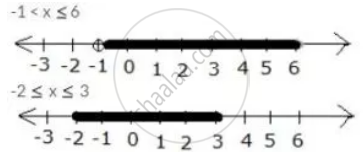Share

# Use the Real Number Line to Find the Range of Values of X for Which: -1 < X ≤ 6 and -2 ≤ X ≤ 3 - Mathematics

Course

#### Question

Use the real number line to find the range of values of x for which:

-1 < x ≤ 6 and -2 ≤ x ≤ 3

#### Solution

-1 < x ≤ 6 and -2 ≤ x ≤ 3

Both the given inequations are true in the range where their graphs on the real number lines overlap.

The graphs of the given inequations can be drawn as:From both graphs, it is clear that their common range is 1 < x ≤ 3

Is there an error in this question or solution?